# SSC CHSL Practice Paper

### Section 3: Mathematics : SSC CHSL Practice Paper

Q.1: A rhombus of side 28 cm has one angle of 60o. What is the length of the larger diagonal ?
(A)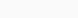$28(1+\sqrt3)$ cm
(B)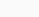$28\sqrt2$ cm
(C) 28 cm
(D)$28\sqrt3$ cm

Ans : (D)$28\sqrt3$ cm

Q.2: if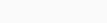$\sqrt x+\frac{1}{\sqrt x}=\sqrt6$, then the value of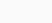$x^6+\frac {1}{x}^6$ will be:
(A) 2270
(B) 2502
(C) 2702
(D) 2712

Ans : (C) 2702

Q.3: An article was sold for ₹512 after giving two equal successive discounts on the marked price of ₹800. the percentage discount offered is:
(A) 16%
(B) 20%
(C) 18%
(D) 15%

Ans : (B) 20%

Q.4: Varun and Madhur invested ₹25,000 each in different schemes. Varun earned simple interest at 11% per annum, whereas Madhur earned compound interest at 10% per annum compounded annually. Who received more interest after 2 years and how much ?
(C) Varun, ₹500
(D) Varun, ₹250

Ans : (D) Varun, ₹250

Q.5: Study the given pie chart and answer the question that follows.
The pie chart shows the distribution (degree-wise) of the number of computers sold by a shopkeeper during five months.
total number of Computers Sold = 5400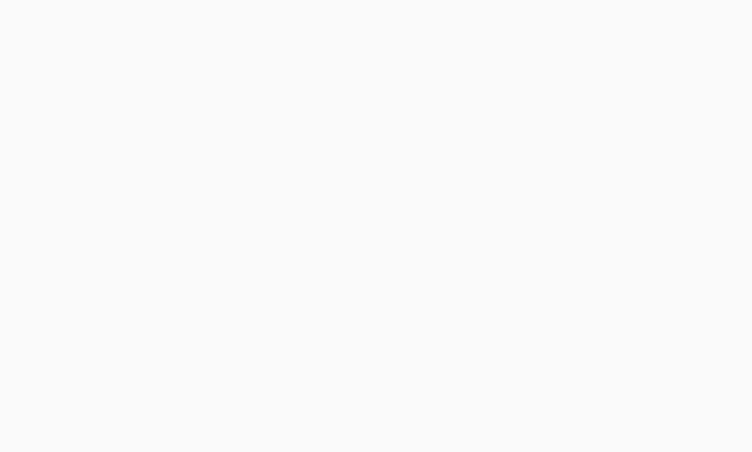In which month was the number of computers sold above 25% of the total number of computers sold in 5 months?

(A) March
(B) May
(C) February
(D) January

Ans : (A) March

Q.6: ABC is a right-angled triangle, right-angled at B. D is a point on AC such that AD = 12 cm and CD=16cm. If BD bisects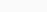$\angle\text{ABC}$ ,then the perimeter of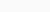$\triangle\text{ABC}$ will be:
(A) 66 cm
(B) 56.2 cm
(C) 66.2 cm
(D) 67.2 cm

Ans : (D) 67.2 cm

Q.7: The average daily income of Shyam Lal during the month of February 2020 was ₹560. The average income for the first 16 days was ₹590 and for the last 16 days it was ₹500. what was his average income for 14th ,15th and 16th February?
(A) ₹545
(B) ₹400
(C) ₹590
(D) ₹587

Ans : (B) ₹400

Q.8: A train starts running at a uniform speed of 60 km h from station P towards station Q. At the same time another train starts running from station Q towards station P. If the distance between the stations P and Q is 275 km and the trains meet in two and a half hours, then what is the speed of the train running towards station P in km h?
(A) 48
(B) 44
(C) 40
(D) 50

Ans : (D) 50

Q.9: Two chords AB and CD of a circle intersect at O. If CO=4 cm, OD=3.75 cm, and AB=8 cm, then what is the length (in cm) of the smaller among AO and OB?
(A) 2.75
(B) 5.25
(C) 5
(D) 3

Ans : (D) 3

Q.10: A is thrice as efficient as as B. A takes 24 days less than B to complete a work. If they work together, in how many days, will the work be completed?
(A) 9
(B) 12
(C) 15
(D) 8

Ans : (A) 9

Q.11: If a number 54k 31m 82 is divisible by 11, what will be the maximum value of (k+m)?
(A) 23
(B) 13
(C) 12
(D) 11

Ans : (B) 13

Q.12: If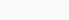$x-\frac {2}{4}=4$, then what will be the value of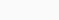$x^2+\frac {4}{x^2}?$
(A) 20
(B) 18
(C) 12
(D) 8

Ans : (A) 20

Q.13: In triangle ABC, if BD and CD bisect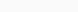$\angle\text{B and}\angle\text{C}$ , respectively, and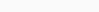$\angle\text{BDC}=135^o$, then find the measure of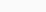$\angle\text{BAC}$ ,
(A) 90o
(B) 75o
(C) 45o
(D) 65o

Ans : (A) 90o

Q.14: In a circle with centre O, PA and PB are the tangents at A and B, respectively, from an external point P, If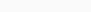$\angle\text{APB}=42^o$ , then what will be the measure of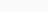$\angle\text{AOB}$?
(A) 121o
(B) 142o
(C) 159o
(D) 138o

Ans : (D) 138o

Q.15: The greatest number among 350, 440, 530 and 620 is
(A) 350
(B) 440
(C) 530
(D) 620

Ans : (B) 440

Q.16: The number of students enrolled in different streams at senior Secondary level in five schools has been shown in the bar graph.
What is the ratio of the number of students enrolled in the vocational stream in schools A, C and E taken together to those enrolled in the humanities stream in the schools B, D and E taken together ?

(A) 5 : 6
(B) 7 : 9
(C) 2 : 3
(D) 9 : 11

Ans : (A) 5 : 6

Q.17: if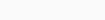$x^4+\frac{1}{x^4}=3842$ , then the positive value of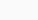$x+\frac1x$ will be:
(A) 12
(B) 8
(C) 10
(D) 6

Ans : (B) 8

Q.18: The value of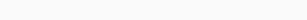$31\frac25\div[168\div\frac37of28+(33\div\frac52)+(7\frac35-3\frac25)]$ is:
(A) 5
(B) 1
(C) 3
(D) 10

Ans : (B) 1

Q.19: If cosec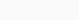$\theta=\frac{41}{9} \text{and}\theta$ is an acute angle, then the value of 5 tan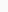$\theta$ will be:
(A)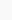$\frac{11}{8}$
(B)$\frac{13}{4}$
(C)$\frac{7}{8}$
(D)$\frac{9}{8}$

Ans : (D)$\frac{9}{8}$

Q.20: The volume of a metallic cylindrical pipe is 3564 cm3 .If its external radius is 12 cm and thickness is 3 cm, then the length of the pipe will be:
(take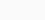$\pi = \frac{22}{7}$)
(A) 22 cm
(B) 20 cm
(C) 18 cm
(D) 16 cm

Ans : (C) 18 cm

Q.21: A shopkeeper bought pens at the rate of ₹1,350 for 15 dozen pens and sold them at the rate of ₹495 for 5 dozen pens. His percentage profit or percentage loss is:
(A) Profit 10%
(B) Profit 15%
(C) Loss 15%
(D) Loss 10%

Ans : (A) Profit 10%

Q.22: Study the following graph and answer the question that follows.
Marks scored by five students (A, B, C, D and E) in three subjects (Maths, English and Physics)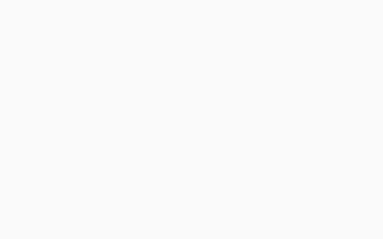What is the ratio of the marks of D’s in Maths and Physics together to the marks of B’s in English and Physics?

(A) 14 : 13
(B) 7 : 14
(C) 9 : 14
(D) 2 : 7

Ans : (C) 9 : 14

Q.23: The population of a town increased by 15% in 2019. Due to a pandemic, it decreased by 10% in 2020. What was the percentage increase in the population of the town in 3 years?
(A) 12.5%
(B) 17.5%
(C) 13.85%
(D) 15%

Ans : (C) 13.85%

Q.24: If a : b =2 : 3 and c : d = 5a : 3b, then 2c : 5d is equal to:
(A) 1 : 1
(B) 10 : 9
(C) 4 : 9
(D) 9 : 10

Ans : (C) 4 : 9

Q.25: Solve the following equation and find the value of$\theta$.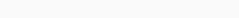$3cot\theta+tan\theta-2\sqrt3=0,0 \angle\theta\angle90^o$
(A) 30o
(B) 45o
(C) 60o
(D) 15o